# Natural Language Processing¶

In [ ]:
%%capture
from ipypublish import nb_setup


## Introduction¶

Natural Language Processing or NLP is an important application area for Neural Networks. Just as images are full of patterns in pixel space which can detected by Neural Networks, human language also presents a rich panorama of patterns which Neural Networks can exploit. In order to detect these patterns, words and sentences have to coded and then fed into the model. We start by describing how this is done in the section on Word Embeddings. It is shown there that words can be represented using 1-D tensors or vectors, the contents of which are analogous to the RGB pixels in an image. These vectors, called Word Embeddings, are arranged in N dimensional vector space in a way such that words with similar meanings have their embeddings cluster together. Once we know how to embed words, the next step is to encode entire sentences or even larger units. In order to do this we use the Neural Network models that we have developed in the last few chapters, in particular RNNs and LSTMs (including GRUs). The process of finding representations for a sequence of words is called Language Modeling and we show how this can be accomplished using Self-Supervised Learning. Once we have such a representation, it can be used for a variety of useful tasks, including:

• Machine Translation
• Text Categorization
• Speeech Transcription
• Text Summarization

Since NLP is an commercially important technology, decades of work had gone into it the pre-Deep Learning era. Within a few short years, Neural Network models have begun to equal or better older techniques in tasks such as Speech Transcription and Machine Translation, and steady progress is being made in other areas as well.

In this chapter we will make extensive use of Neural Network models as Generators, i.e., they will be used to generate sentences (in contrast to earlier chapters in which Neural Networks were used mainly for classification). Generative Modeling is an emerging area in Deep Learning, and has also been used to generate images as shown in Chapter ConvNetsPart2 and in the chapter on Unsupervised Learning. However unlike for Image Processing, Generative Modeling is integral to performing important NLP applications such Machine Translation and Speech Transcription.

In the next chapter we will introduce a class of Neural Network models called Transformers that were introduced recently (in 2017). They constitute another way of modeling sequences, beyond RNNs and LSTMs, and outperform these older models in most NLP tasks. Transformers can also be pre-trained and then used do Transfer Learning for NLP tasks, just like ConvNets are used for Transfer Learning for images. This is an exciting emerging area which is still in its research phase.

## Word Embeddings¶

The coding rule used to encode words in a text document is known as Word Embedding and it is used to convert a vocabulary of words into points in a high dimensional co-ordinate space. In previous chapters we used Word Embeddings to encode the text in the IMDB Movie Review dataset, and the reader may recall that there were two ways in which it could be done:

1. By using pre-trained Word Embeddings, such as Word2Vec or Glove
2. By using Gradient Descent to find the embeddings as part of the model training process

Method 1 works better if the training data is limited, since the Word Emveddings are already pre-trained on a much larger vocabulary. If there is enough data available then Method 2 should be tried, since it learns embeddings that are more appropriate for the problem at hand. In this section we focus on Method 1 and show how pre-training is done for the Word2Vec model.

The most straightforward rule for embedding is the 1-of-K Encoding (also known as One-Hot Encoding), and works as follows: If the vocabulary under consideration consists of a certain number of words, say 20,000 words, then the system encodes them using a 20,000 dimensional vector, in which all the co-ordinates are zero, except for the index co-ordinate for the word, that is set one. There are two problems with this scheme:

• It results in extremely high dimensional input vectors, and the dimension increases with increasing number of words in the vocabulary.
• The coding scheme does not incorporate any semantic information about the words, since the representations are all located equi-distant from each other at the vertices of a K-dimensional cube.

In order to remedy these problems, researchers have come up with several alternative algorithms to do Word Embeddings, one of the most popular of which is Word2Vec. This representation is created by making use the words that occur frequently in the neighborhood of the target word, and it has been shown to capture many linguistic regularities.

In [ ]:
#rnn50
nb_setup.images_hconcat(["DL_images/rnn50.png"], width=1000)

Out[ ]: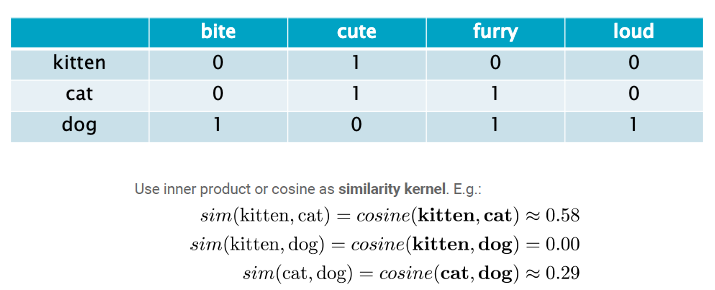In order to understand how the algorithm works, consider the table in Figure rnn50 which considers the problem of encoding the words "kitten", "cat" and "dog". In order to capture the meaning of these three words, we represent them using a vector of size four as shown. The co-ordinates of this vector encode the following characteristics which are found in the original three words: bite, cute, furry and loud. For example a kitten can be characterized as being cute, while a cat is furry and loud in addition to being cute. Representing of words using this vector scheme captures semantic information about them, and it is concievable that words that are closer in meaning will have vectors that occur closer to each other. One way of capturing this similarity is by using the inner product or cosine between the vectors, and as shown above, by this measure cats and kittens are more similar as compared to dogs and kittens.

Word2Vec encodes words into vectors by automating the procedure that was described above. Instead of having to manually specify the characteristics of a word, Word2Vec automatically infers this information by other words that occur frequently together in the same sentence as the target word (for example sentences that have the words "kitten" and "cute" would occur more frequently than sentences with the words "kitten" and "bite").

In [ ]:
#CBOW
nb_setup.images_hconcat(["DL_images/rnn28.png"], width=1000)

Out[ ]: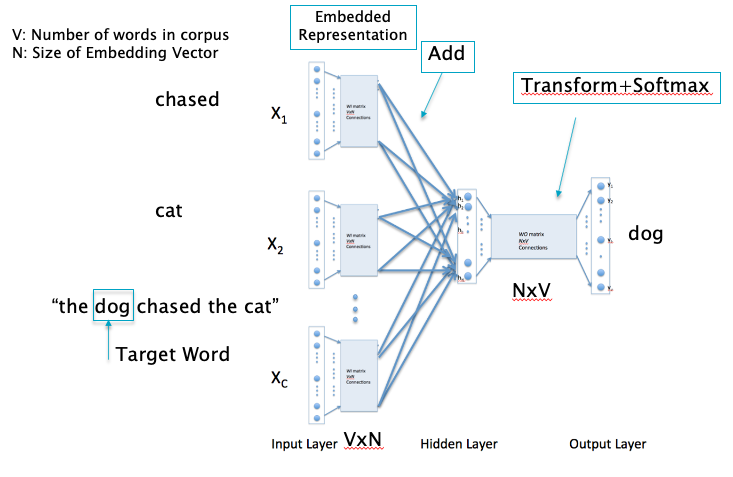Word2Vec uses two algorithms to generate Word Embeddings, namely Continuous Bag of Words (CBOW) and Skip-Gram. Both these algorithms use shallow Neural Networks that are trained in a self-supervised manner, so they are extremely fast. Figure CBOW shows the CBOW network, and it works as follows: The Input Layer consists of 1 of K encoded representations for all the words in a sentence, except for the target word. In the example shown, for the sentence "the dog chased the cat", the target word is set to "dog" and the words "chased" and "cat" are the inputs into the network. The main idea of the algorithm is to train the network with backprop while using the target word as the label, and this is done in two linear stages:

• In the first stage each of the 1-Hot-Encoded word vectors (which are of size V, where V is the number of words in the vocabulary) are transformed into a smaller vector of size N, where N is the dimension of the embedding, by using a matrix multiplication (the same matrix parameters are used for all the inputs).
• In the second stage, the results of the first stage are added together to form a vector of size N, and then passed through another matrix multiplication into V output nodes, the contents of which are sent through a softmax layer to generate the classification probabilities.

This network is trained using all the sentences in the corpus, with each word in a sentence taking turns as the target word. Note that this is an example of Self-Supervised Learning, since the labels are generated automatically using the input dataset itself. Once the network is fully trained, the first stage of the network serves as the transform to generate an embedding.

In [ ]:
#Skip-Gram
nb_setup.images_hconcat(["DL_images/rnn49.png"], width=1000)

Out[ ]: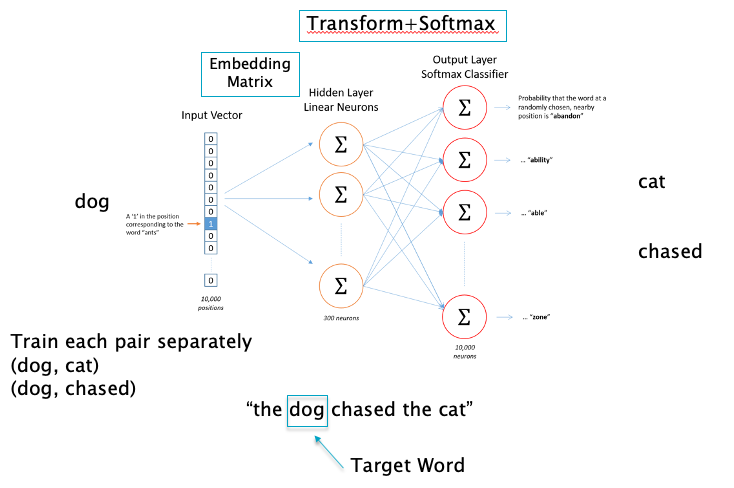The operation of the Skip-Gram network, shown in Figure Skip-Gram, is some ways the inverse of the CBOW network. As we saw earlier, CBOW tries to predict a target word by making use of the context provided by the surrounding words. On the other hand, given a sentence, Skip-Gram tries to predict the surrounding words with the target word now serving as an input into the network. This is illustrated in the figure using the sentence "The dog chased the cat": The word "dog" is input into the Skip-Gram network while the words "cat" and "chased" serve as target labels for training the network. Note that this is an example of multi-label classification since the network is being used to do multiple classifications based on a single input. The network itself is a simple Dense Feedforward network with a single Hidden Layer and two transform stages, just as for the CBOW network. The transform carried out in the first stage serves as the Embedding Matrix, while the second stage transform is used to generate the Logit Values for classification.

In [ ]:
#rnn51
nb_setup.images_hconcat(["DL_images/rnn51.png"], width=600)

Out[ ]: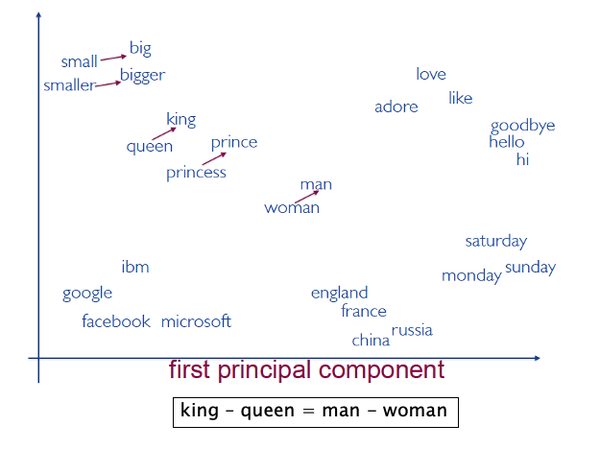In general words that are semantically close to each other tend to cluster together in the embedded space as shown in FIgure rnn51. As an example, consider the following vector operation which makes use of Word Embeddings (vec('x') stands for the Word Embedding representation for the word 'x'):

$$vec('Paris') - vec('France') = vec('Rome') - vec('Italy')$$

Hence Word Embeddings incorporate the meaning of word into their representations, which gives better results when these vectors used in various operations involving words and language.

It should also be noted that instead of encoding words, we could encode at the character level instead. The benefit of doing this is that the number of characters in a dataset are limited to a few hundred at most, while the number of words can easily number in the tens of thousands. The tradeoff is that there is less semantic structure at the character level so that model performance may not be optimun). Hence 1 of K encoding is almost always the default choice for character level embedding.

## Text Classification¶

In [ ]:
#rnn73
nb_setup.images_hconcat(["DL_images/rnn73.png"], width=800)

Out[ ]: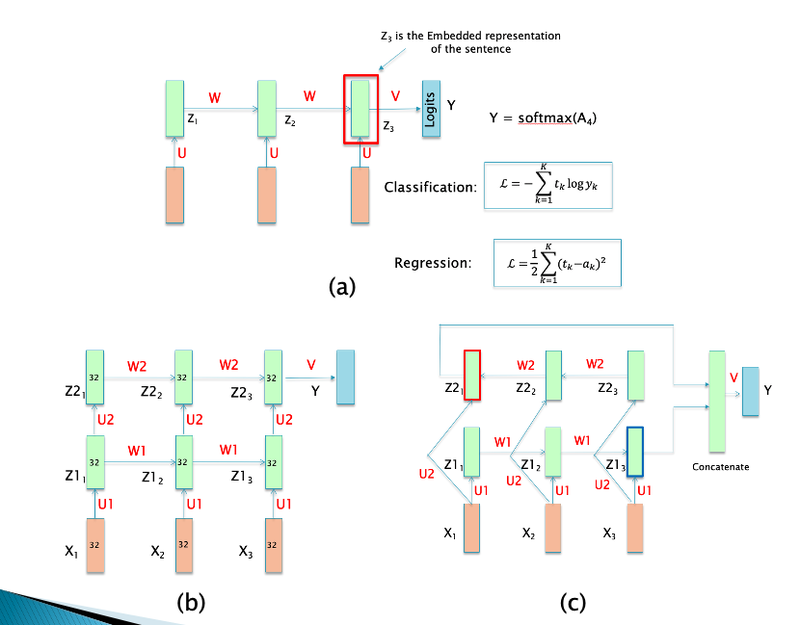Text Classification is an important applications of NLP. Here is a list of ways in which it can be used:

• Detecting spam emails
• Sentiment Analysis: For example classifying investor sentiment (Buy or Sell) based on classification of news articles or tweets
• Classifying the topic of an article based on its text
• Classifying the language for an article
• Classifying the author of an article

The chapter on RNNs contains several examples of techniques for doing text classification, some of which are shown in Figure rnn73. Part (a) of this figure shows the most straightforward way for doing text classification, while (c) (c) show more complex networks which can improve the accuracy, either by incorporating multiple layers as in (b) or a bi-directional architecture as in (c). The latter two network types can also be combined easily with Keras into a multi-layer bi-directional RNN.

In [ ]:
#rnn74
nb_setup.images_hconcat(["DL_images/rnn74.png"], width=600)

Out[ ]: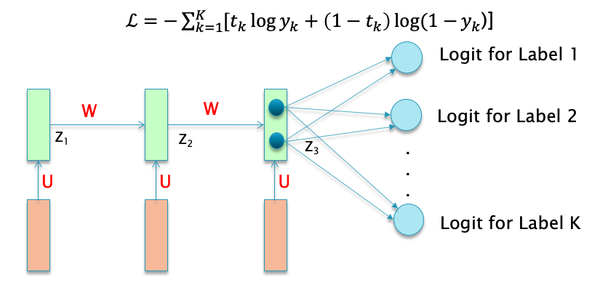Figure rnn74 shows an example of multi-label text classification using a multi-headed RNN. Note that we are trying to classify into just two categories for each class, i.e., present or not-present, hence we use Binary Cross Entropy Loss function for each. These are then aggregated across all the categories to obtain the final Loss Function as shown in the figure.

The following example, taken from Chollet Section 7.1.3, shows an application of multi-label classification applied to tweet inputs to predicting the following about the tweeter:

1. Age: This is predicted using Mean Square Error based regression, hence the MSE Loss Function
2. Income: This is classified into a number of discrete categories, hence uses the Categorical Cross Entrop Loss Function
3. Gender: Since there are only 2 categories, we use the Binary Cross Entropy Loss Function
In [ ]:
#rnn75
nb_setup.images_hconcat(["DL_images/rnn75.png"], width=800)

Out[ ]: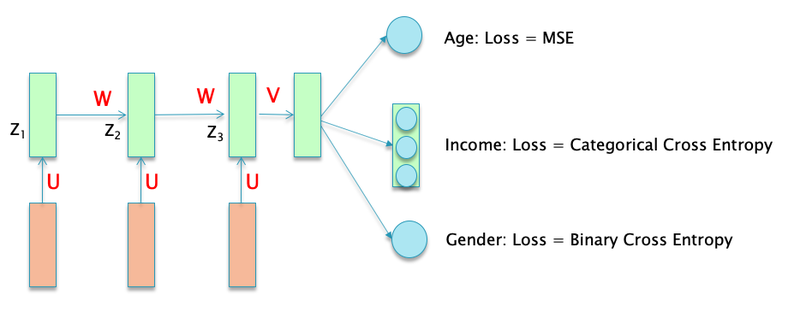In order to code the multi-headed model, we use the Keras Functional API. Each of the three prediction types gets its own Logit Layer. Note that since the Age prediction is being done using Regression, only a single node is needed for its Logit.

In [ ]:
from keras import layers
from keras import Input
from keras.models import Model

vocabulary_size = 50000
num_income_groups = 10
posts_input = Input(shape=(None,), dtype='int32', name='posts')
embedded_posts = layers.Embedding(256, vocabulary_size)(posts_input)

x = layers.SimpleRNN(32)(embedded_posts)
x = layers.Dense(128, activation='relu')(x)

age_prediction = layers.Dense(1, name='age')(x)
income_prediction = layers.Dense(num_income_groups, activation='softmax', name='income')(x)
gender_prediction = layers.Dense(1, activation='sigmoid', name='gender')(x)

model = Model(posts_input, [age_prediction, income_prediction, gender_prediction])


Even though each output type gets its own loss function, all three of them need to be combined together into a singe scalar value in order to do Gradient Descent optimization. This can be done during model compilation as shown below. Since the three Loss Functions can differ in values by quite a bit, it is recommended that their values be appropriately weighted, which can be done using the loss_weights field. The MSE Loss Function assumes values around 3-5 while the Binary Cross Entropy Loss Function assumes values which are can be less than 1, which justifies the weights shown in the example.

In [ ]:
model.compile(optimizer='rmsprop',
loss={'age': 'mse',
'income': 'categorical_crossentropy',
'gender': 'binary_crossentropy'},
loss_weights={'age': 0.25,
'income': 1.,
'gender': 10.})


Assuming that model input posts and the outputs age_targets, income targets and gender_targets are already in the form of Numpy arrays, they can be passed on to the fit command as shown.

In [ ]:
model.fit(posts, {'age': age_targets,
'income': income_targets,
'gender': gender_targets},
epochs=10, batch_size=64)


In the following example we use an LSTM model to classify the movie reviews in the IMDB dataset. We start by invoking the text_dataset_from_directory function to create the training, validation and test samples.

In [ ]:
import os, pathlib, shutil, random
from tensorflow import keras
batch_size = 32
base_dir = '/Users/subirvarma/handson-ml/datasets/aclImdb'

train_ds = keras.utils.text_dataset_from_directory(
"/Users/subirvarma/handson-ml/datasets/aclImdb/train", batch_size=batch_size
)
val_ds = keras.utils.text_dataset_from_directory(
"/Users/subirvarma/handson-ml/datasets/aclImdb/val", batch_size=batch_size
)
test_ds = keras.utils.text_dataset_from_directory(
"/Users/subirvarma/handson-ml/datasets/aclImdb/test", batch_size=batch_size
)
text_only_train_ds = train_ds.map(lambda x, y: x)

Found 20000 files belonging to 2 classes.
Found 5000 files belonging to 2 classes.
Found 25000 files belonging to 2 classes.


The TextVectorization in invoked to convert the text into integers, for each sample review. Each review is restricted to 600 characters or less, and the vocabulary used is restricted to the 20,000 most frequently ocurring words.

In [ ]:
from tensorflow.keras import layers

max_length = 600
max_tokens = 20000
text_vectorization = layers.TextVectorization(
max_tokens=max_tokens,
output_mode="int",
output_sequence_length=max_length,
)

int_train_ds = train_ds.map(lambda x, y: (text_vectorization(x), y))
int_val_ds = val_ds.map(lambda x, y: (text_vectorization(x), y))
int_test_ds = test_ds.map(lambda x, y: (text_vectorization(x), y))


The main model is a single layer LSTM with 32 nodes per cell.

In [ ]:
vocab_size = 20000
embed_dim = 32

inputs = keras.Input(shape=(None,), dtype="int64")
x = layers.Embedding(vocab_size, embed_dim)(inputs)

x = layers.LSTM(32)(x)
outputs = layers.Dense(1, activation='sigmoid')(x)
model = keras.Model(inputs, outputs)
model.compile(optimizer="rmsprop",
loss="binary_crossentropy",
metrics=["accuracy"])
model.summary()

Model: "model_6"
_________________________________________________________________
Layer (type)                 Output Shape              Param #
=================================================================
input_6 (InputLayer)         [(None, None)]            0
_________________________________________________________________
embedding_6 (Embedding)      (None, None, 32)          640000
_________________________________________________________________
lstm_5 (LSTM)                (None, 32)                8320
_________________________________________________________________
dense_6 (Dense)              (None, 1)                 33
=================================================================
Total params: 648,353
Trainable params: 648,353
Non-trainable params: 0
_________________________________________________________________

In [ ]:
history = model.fit(int_train_ds, validation_data=int_val_ds, epochs=15)

Epoch 1/15
625/625 [==============================] - 143s 224ms/step - loss: 0.6935 - accuracy: 0.4949 - val_loss: 0.6932 - val_accuracy: 0.5000
Epoch 2/15
625/625 [==============================] - 133s 212ms/step - loss: 0.6922 - accuracy: 0.5025 - val_loss: 0.6875 - val_accuracy: 0.5124
Epoch 3/15
625/625 [==============================] - 134s 215ms/step - loss: 0.6851 - accuracy: 0.5102 - val_loss: 0.6977 - val_accuracy: 0.5084
Epoch 4/15
625/625 [==============================] - 134s 215ms/step - loss: 0.6817 - accuracy: 0.5163 - val_loss: 0.6873 - val_accuracy: 0.5086
Epoch 5/15
625/625 [==============================] - 133s 213ms/step - loss: 0.6706 - accuracy: 0.5787 - val_loss: 0.6773 - val_accuracy: 0.6232
Epoch 6/15
625/625 [==============================] - 133s 213ms/step - loss: 0.6098 - accuracy: 0.6816 - val_loss: 0.6522 - val_accuracy: 0.6336
Epoch 7/15
625/625 [==============================] - 133s 212ms/step - loss: 0.5918 - accuracy: 0.7066 - val_loss: 0.5835 - val_accuracy: 0.7388
Epoch 8/15
625/625 [==============================] - 133s 213ms/step - loss: 0.5767 - accuracy: 0.7246 - val_loss: 0.6021 - val_accuracy: 0.7166
Epoch 9/15
625/625 [==============================] - 134s 215ms/step - loss: 0.5742 - accuracy: 0.7232 - val_loss: 0.5960 - val_accuracy: 0.7408
Epoch 10/15
625/625 [==============================] - 138s 221ms/step - loss: 0.5820 - accuracy: 0.7329 - val_loss: 0.5793 - val_accuracy: 0.7436
Epoch 11/15
625/625 [==============================] - 138s 220ms/step - loss: 0.6003 - accuracy: 0.6848 - val_loss: 0.7171 - val_accuracy: 0.5056
Epoch 12/15
625/625 [==============================] - 137s 220ms/step - loss: 0.5919 - accuracy: 0.6920 - val_loss: 0.5772 - val_accuracy: 0.7496
Epoch 13/15
625/625 [==============================] - 138s 221ms/step - loss: 0.5871 - accuracy: 0.7046 - val_loss: 0.6061 - val_accuracy: 0.7044
Epoch 14/15
625/625 [==============================] - 138s 220ms/step - loss: 0.5708 - accuracy: 0.7199 - val_loss: 0.6384 - val_accuracy: 0.6780
Epoch 15/15
625/625 [==============================] - 138s 220ms/step - loss: 0.5928 - accuracy: 0.7007 - val_loss: 0.5868 - val_accuracy: 0.7408


## Language Models¶

Language Modeling is a fundamental task in NLP. It enables the NLP model to learn the underlying statistical structure of a language, which then enables it to generate valid sentences that are gramatically correct and are likely to have been spoken in that language. Language generation based on Language Models is very powerful tool that has been put to use in a number of commercially important applications such as Machine Translation, Speech Transcription etc.

There are two equivalent definitions of a Language Model that we find in the literature:

1. Given a sequence of words $(w_1,...,w_N)$, a Language Model can be used to generate the most probable next word $w_{N+1}$ in the sequence.
2. Given a sequence of words $(w_1,...,w_N)$, a Language Model can be used to compute the probability $p(w_1,...,w_N)$ of that sequence occuring in the language (see Figure rnn52).
In [ ]:
#rnn52
nb_setup.images_hconcat(["DL_images/rnn52.png"], width=600)

Out[ ]: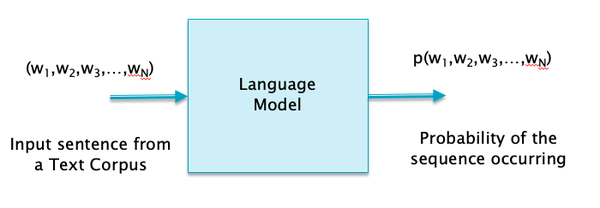Figure rnn53 shows an application of the first definition of Language Models that we see on an everyday basis, i.e., search engines with the ability to automatically generate the next few words in the search term before they are typed. Another common application is the Auto Reply feature for emails.

In [ ]:
#rnn53
nb_setup.images_hconcat(["DL_images/rnn53.png"], width=600)

Out[ ]: